Multiple Choice
Identify the choice that best completes the statement or answers the question.

1.

Measurement – Length, Weight, Volume – RIT 211 – 220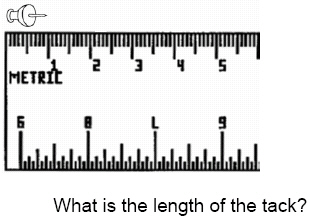a. 1 cm d. 1 m b. 2 cm e. 1 mm c. 5 cm

2.

Measurement – Money – RIT 211 – 220
If Jamaal will be paid \$5.00 an hour for delivering pizzas, and he works for
4 hours, how much will he be paid?
 a. \$9.00 d. \$2.00 b. \$5.00 e. \$20.00 c. 20

3.

Measurement – Area, Perimeter, Circumference – RIT 221 – 230a. AC d. Point D b. BC e. E. Arc E c. BD

4.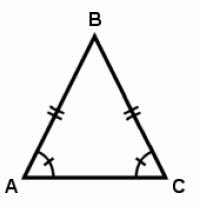What is the measure of angle B IF this is an equilateral triangle?
 a. 1200 d. 1800 b. 500 e. 300 c. 600

5.

Measurement – Length, Weight, Volume – RIT 221 – 230a. 5 cm d. 4 cm b. 5 mm e. 4 mm c. 3 cm

6.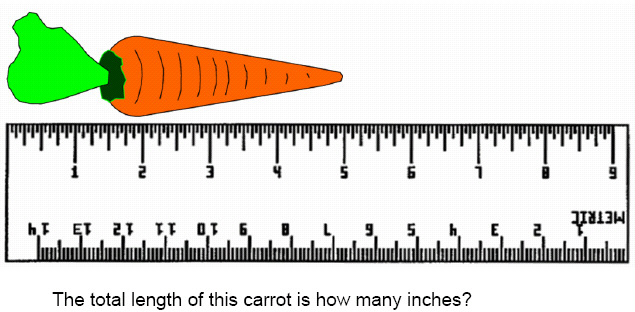a. 5.5” d. 5 centimeters b. 5” e. 5’ c. 5 yds.

7.

If you were asked to calculate the length of a football field, would you use
yards, quarts, inches, centimeters, or miles?
 a. quarts d. centimeters b. inches e. miles c. yards

8.

Number Sense and Numeration – Decimals – RIT 211 – 220

Round the following to the nearest cent: \$16.997
 a. \$16.99 d. \$16.00 b. \$16.10 e. \$20.00 c. \$17.00

9.

Which of the following fractions would become a repeating decimal?
 a. 1/8 d. 7/9 b. 3/4 e. 5/8 c. 27/36

10.

Number Sense and Numeration – Exponents – RIT 211 – 220
3,257 equals:
 a.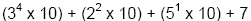d.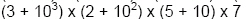b.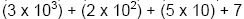e.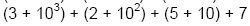c.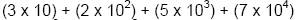11.

Express in scientific notation: 215,000,000
 a.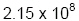d.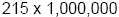b.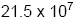e.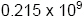c.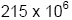12.

Express the following number in standard form: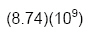a. 874,000 d. 8,740,000,000 b. 8,741,000,000 e. 874,000,000 c. 874,000,000,000

13.

Number Sense and Numeration – Fractions – RIT 211 – 220

Express the following as an improper fraction: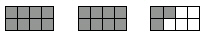a. 2 3/8 d. 19/24 b. 23/8 e. 5/8 c. 19/8

14.

What is the simplest form of 27/42?
 a. 27/42 d. 9/14 b. 1 15/27 e. 13/21 c. 2/3

15.

Number Sense and Numeration – Ordering, Equalities and Inequalities –
RIT 211 – 220

Order the following fractions from least to greatest:
4/8, 1/4, 4/6, 2/6
 a. 1/3, 2/3, 1/4, 2/4 d. 1/4, 2/6, 4/6, 4/8 b. 4/6, 4/8, 2/6, 1/4 e. 1/4, 2/6, 4/8, 4/6 c. 1/2, 1/3, 1/4, 2/3

16.

Which of the following is a true statement?
 a.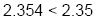d.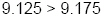b.e.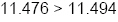c.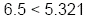17.

Number Sense and Numeration – Percents – RIT 211 – 220

Express 1/8 as both a decimal and a percent.
 a. 0.18 = 18% d. 0.125 = 12.5% b. 0.125 = 125% e. 0.13 = 13% c. 1.25 = 12.5%

18.

Number Sense and Numeration – Whole Numbers – RIT 211 – 220

Determine whether 42 is divisible by 2, 3, 5, 6, and/or 10.
 a. 2 d. 2, 3, 5, 6 b. 2, 3 e. 2, 3, 5, 10 c. 2, 3, 6

19.

The greatest common factor of 54 and 72 is:
 a. 9 d. 2 b. 18 e. 6 c. 1

20.

Which are the greatest common factor and the least common multiple of the
numbers 12 and 9?
 a. G.C.F. = 3; L..C.M. = 24 d. G.C.F. = 1; L.C.M. = 108 b. G.C.F. = 3; L.C.M. = 36 e. G.C.F. = 3; L.C.M. = 27 c. G.C.F. = 6; L.C.M. = 108